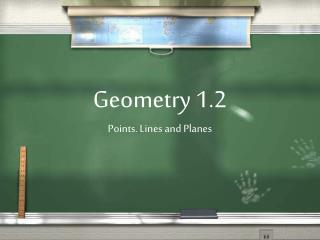DownloadDownload PresentationGeometry 1.2 Points. Lines and Planes

# Geometry 1.2 Points. Lines and Planes

Download Presentation## Geometry 1.2 Points. Lines and Planes

- - - - - - - - - - - - - - - - - - - - - - - - - - - E N D - - - - - - - - - - - - - - - - - - - - - - - - - - -
##### Presentation Transcript

1. Geometry 1.2Points. Lines and Planes

2. Points • Basic Unit of Geometry • Has no size • Named using capital letters • Points are written as capital letters A, B, C

3. What Points Look Like A S B M R

4. If we get a lot of points together and they are straight we make a line! B C S P M L

5. Lines • Are made of an infinite number of points • Extend in both directions FOREVER • Named by two points on the line • Or with a lowercase cursive letter • The name is written with a symbol AB SV KT

6. What Lines Look Like d S M G

7. Vocabulary • Collinear = • points on the same line • Noncollinear = • Not on the same line

8. Collinear/Noncollinear d S l H M Q G

9. Rays • Are lines that end on one side • Extend in one direction FOREVER • Named by the endpoint and one other point on the ray • The name is written with a symbol CB KM UV

10. What do rays look like V K M C U V X

11. Line Segment • The chunk of line between two points • Could be part of a line or ray or alone • Named by the endpoints • The name is written with a symbol BH DG PV

12. What do Line segments look like V K M C U V X

13. Plane • Flat surface • Extends FOREVER • Named by three points in the plane • Or by an uppercase cursive letter • The name is written like this ABC JKM R

14. More Vocabulary • Coplanar = • points on the same • Noncoplanar = • Not on the same plane plane

15. What do planes look like R V M K C U

16. 1.23-27